Courses

# RD Sharma Solutions -Page No.5.9, Negative Numbers And Integers, Class 6, Maths Class 6 Notes | EduRev

## RD Sharma Solutions for Class 6 Mathematics

Created by: Abhishek Kapoor

## Class 6 : RD Sharma Solutions -Page No.5.9, Negative Numbers And Integers, Class 6, Maths Class 6 Notes | EduRev

The document RD Sharma Solutions -Page No.5.9, Negative Numbers And Integers, Class 6, Maths Class 6 Notes | EduRev is a part of the Class 6 Course RD Sharma Solutions for Class 6 Mathematics.
All you need of Class 6 at this link: Class 6

#### PAGE NO 5.9:

Question 1:Draw a number line and represent each of the following on it:

(i) 5+(−2)5+-2

(ii) (−9)+4-9+4

(iii) (−3)+(−5)-3+-5

(iv) 6+(−6)6+-6

(v) (−1)+(−2)+2-1+-2+2

(vi) (−2)+7+(−9)

ANSWER: (i) If we start from 5 and move 2 units to the left of 5, we will obtain 3, as shown on the number.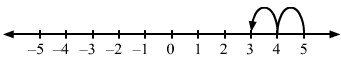(ii) If we start from -9 and move 4 units to the right of -9, we will obtain -5, as shown on the number line.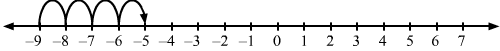(iii) If we start from -3 and move 5 units to the left of -3, we will obtain -8, as shown on the number line.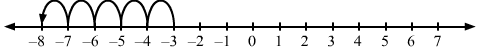(iv) If we start from 6 and move 6 units to the left of 6, we will obtain 0, as shown on the number line.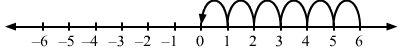(v) If we start from -1 and move 2 units to the left of -1, we will obtain -3; and then if we start from -3 and move 2 units to the left, we will obtain -1, as shown on the number line.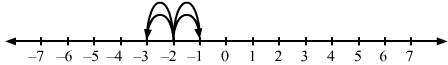(vi) If we start from -2 and move 7 units to the right, we will obtain 5; and then if we start from 5 and move 9 units to the left, we will obtain -4, as shown on the number line.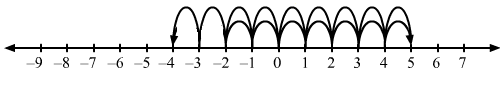103 docs

,

,

,

,

,

,

,

,

,

,

,

,

,

,

,

,

,

,

,

,

,

,

,

,

,

,

,

,

,

,

;